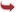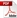International Journal of Scientific and Research Publications

#### IJSRP, Volume 3, Issue 2, February 2013 Edition [ISSN 2250-3153]Mathematical Model by Fuzzy Rules from Dominating Graphs
Dr. G. Nirmala, Mr. K. Dhanabal
Abstract: Mathematical models constructed by fuzzy rules applying the gradient descent method have been widely used in many designs. Adjusting the fuzzy model built for the pre processed data from a dominating graph is the another view point approach of this work. By treating the triangular membership function as two disjoint ones mutual independence of fuzzy rules showed. In that way mathematical models constructed by fuzzy rules by applying gradient descent method with domination graphs.
[VIEW FULL PAPER][DOWNLOAD]

Reference this Research Paper (copy & paste below code):

Dr. G. Nirmala, Mr. K. Dhanabal (2018); Mathematical Model by Fuzzy Rules from Dominating Graphs; Int J Sci Res Publ 3(2) (ISSN: 2250-3153). http://www.ijsrp.org/research-paper-0213.php?rp=P14674